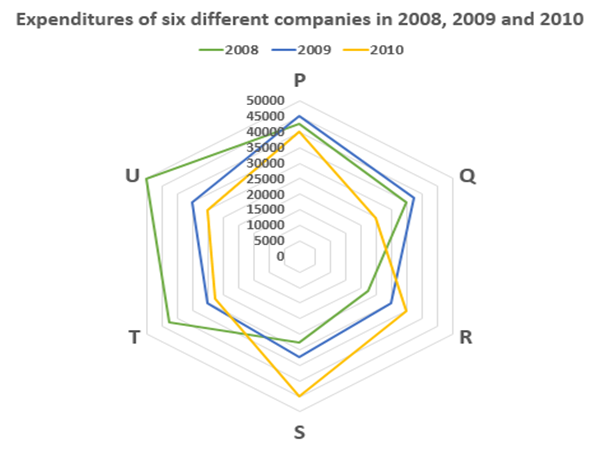# Quantitative Aptitude Questions (Data Interpretation) for SBI Clerk 2018 Day-7

## Quantitative Aptitude Questions (Data Interpretation) for SBI Clerk 2018 Day-7:

Dear Readers, SBI is conducting Online preliminary Examination for the recruitment of Clerical Cadre. preliminary Examination of SBI Clerk was scheduled from March 2018. To enrich your preparation here we have providing new series of Data Interpretation – Quantitative Aptitude Questions. Candidates those who are appearing in SBI Clerk Prelims Exam can practice these Quantitative Aptitude average questions daily and make your preparation effective.

Click “Start Quiz” to attend these Questions and view Solutions

Directions(Q. 1-5) The following data gives year – wise outlay in crores of rupees in a certain 5 year plan (2010-15) of a state. Study the above data carefully and answer the following questions:

 Years Transport Education Housing Health Social welfare 2010-11 15.76% 19.81% 16.26% 16.23% 8.48% 2011-12 20.03% 21.17% 19.15% 17.26% 8.93% 2012-13 20.62% 16.07% 20.12% 18.80% 17.47% 2013-14 21.07% 19.20% 21.08% 20.51% 18.3% 2014-15 22.5% 23.22% 23.37% 27.17% 46.875% total 3564 3806 830 585 224

1. Find the difference between the transport in 2012-13 and social welfare in 2014-15?
1. 840
2. 550
3. 630
4. 130
5. None of these
1. Find the amount of money spent on education in 2013-14, housing in 2012-13 and health in 2010-11?
1. 993
2. 950
3. 731
4. 1067
5. None of these
1. Find the total amount spent in 2011-12?
1. 1800
2. 1600
3. 1700
4. 1500
5. None of these
1. Find the difference between sum of transport in 2010-11 and education in 2014-15 and sum of housing 2010-11 and social welfare in 2013-14?
1. 1465
2. 1180
3. 1270
4. 1265
5. None of these
1. Health sector in 2013-14 is approximately what percentage of 2012-13 in the same outlay?
1. 120%
2. 119%
3. 117%
4. 109%
5. None of these

Directions (Q. 6 – 10): Study the following information carefully and answer the questions given below:1. Expenditures of companies P and Q together in 2008 is what percent of the expenditures of companies T and U in 2009?
1. 116.23%
2. 105.32%
3. 119.23%
4. 117.23%
5. none of these
1. What is the difference between expenditures of all the companies in 2009 and 2010?
1. Rs.7000
2. Rs.7500
3. Rs.8500
4. Rs.8000
5. none of these
1. Find the respective ratio of sum of expenditures of companies P,Q and R in 2009 and that of companies S, T and U in 2010.
1. 35:31
2. 45:41
3. 31:35
4. 41:45
5. none of these
1. Expenditures of companies S and T together in 2008 is what percent less than the expenditures of companies R and S together in 2010?
1. 10.5%
2. 11.5%
3. 12.5%
4. 14.14%
5. none of these
1. If the profit percent of company R in 2009 is 25% and that of company T in 2010 is 18%, find the sum of their incomes.
1. Rs.67940
2. Rs.69850
3. Rs.69950
4. Rs.67950
5. none of these

Directions(Q. 1-5)

The transport in 2012-13=20.62*3564/100=735

Social welfare in 2014-15=46.875*224/100=105

Difference=735-105=630

The education in 2013-14=19.20*3806/100=731

Housing in 2012-13=20.13*830/100=167

Health in 2010-11=16.23/100*585=95

So sum=167+731+95=993

Sum=714+ 806+ 159+ 101 +20=1800

Sum of transport in 2010-11 and education in 2014-15=562+884=1446

Sum of housing 2010-11 and social welfare in 2013-14=135+41=176

Difference=1446-176=1270

Percent=20.5/18.8*100=109%

Directions (Q. 6 – 10):

Expenditures of P and Q together in 2008 = 42500 + 35000 = Rs.77500

Expenditures of T and U together in 2009 = 30000 + 35000 = Rs.65000

Required percentage = 77500/65000 x 100 = 119.23%

Expenditures of all the companies in 2009 = 45000 + 37500 + 30000 + 32500 + 30000 + 35000

= Rs.210000

Expenditures of all the companies in 2010 = 40000 + 25000 + 35000 + 45000 + 27500 + 30000

= Rs.202500

Required difference = 210000 – 202500 = Rs.7500

sum of expenditures of companies P,Q and R in 2009 = 45000 + 37500 + 30000 = Rs.112500

sum of expenditures of companies S,T and U in 2010 = 45000 + 27500 + 30000 = Rs.102500

required ratio = 112500 : 102500 = 45:41

Expenditures of companies S and T together in 2008 = 27500 + 42500 = Rs.70000

Expenditures of companies R and S together in 2010 = 35000 + 45000 = Rs.80000

Required percentage = (80000 – 70000)/80000 x 100 = 12.5%

Income of company R in 2009 = 30000 x 125/100 = Rs.37500

Income of company T in 2010 = 27500 x 118/100 = Rs.32450

Required sum = 37500 + 32450 = Rs.69950